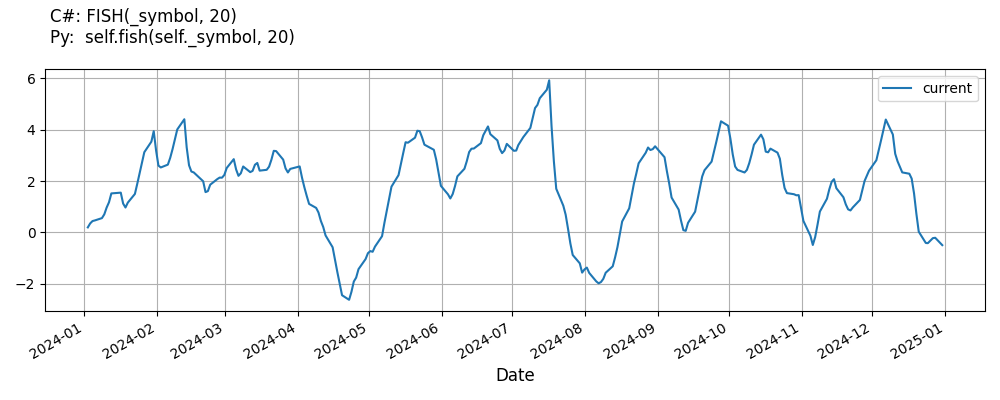# Supported Indicators

## Fisher Transform

### Introduction

The Fisher transform is a mathematical process which is used to convert any data set to a modified data set whose Probability Distribution Function is approximately Gaussian. Once the Fisher transform is computed, the transformed data can then be analyzed in terms of it's deviation from the mean. The equation is y = .5 * ln [ 1 + x / 1 - x ] where x is the input y is the output ln is the natural logarithm The Fisher transform has much sharper turning points than other indicators such as MACD For more info, read chapter 1 of Cybernetic Analysis for Stocks and Futures by John F. Ehlers We are implementing the latest version of this indicator found at Fig. 4 of http://www.mesasoftware.com/papers/UsingTheFisherTransform.pdf

To view the implementation of this indicator, see the LEAN GitHub repository.

### Using FISH Indicator

To create an automatic indicators for FisherTransform, call the FISH helper method from the QCAlgorithm class. The FISH method creates a FisherTransform object, hooks it up for automatic updates, and returns it so you can used it in your algorithm. In most cases, you should call the helper method in the Initialize method.

public class FisherTransformAlgorithm : QCAlgorithm
{
private Symbol _symbol;
private FisherTransform _fish;

public override void Initialize()
{
_fish = FISH(_symbol, 20);
}

public override void OnData(Slice data)
{
{
// The current value of _fish is represented by itself (_fish)
// or _fish.Current.Value
Plot("FisherTransform", "fish", _fish);

}
}
}
class FisherTransformAlgorithm(QCAlgorithm):
def Initialize(self) -> None:
self.fish = self.FISH(self.symbol, 20)

def OnData(self, slice: Slice) -> None:
# The current value of self.fish is represented by self.fish.Current.Value
self.Plot("FisherTransform", "fish", self.fish.Current.Value)



The following reference table describes the FISH method:

### FISH()1/1

            FisherTransform QuantConnect.Algorithm.QCAlgorithm.FISH (
Symbol                            symbol,
Int32                             period,
*Nullable<Resolution>       resolution,
*Func<IBaseData, TradeBar>  selector
)


Creates an FisherTransform indicator for the symbol. The indicator will be automatically updated on the given resolution.

If you don't provide a resolution, it defaults to the security resolution. If you provide a resolution, it must be greater than or equal to the resolution of the security. For instance, if you subscribe to hourly data for a security, you should update its indicator with data that spans 1 hour or longer.

You can manually create a FisherTransform indicator, so it doesn't automatically update. Manual indicators let you update their values with any data you choose.

Updating your indicator manually enables you to control when the indicator is updated and what data you use to update it. To manually update the indicator, call the Update method with a TradeBar, or QuoteBar. The indicator will only be ready after you prime it with enough data.

public class FisherTransformAlgorithm : QCAlgorithm
{
private Symbol _symbol;
private FisherTransform _fish;

public override void Initialize()
{
_fish = new FisherTransform(20);
}

public override void OnData(Slice data)
{
if (data.Bars.TryGeValue(_symbol, out var bar))
{
_fish.Update(bar);
}

{
// The current value of _fish is represented by itself (_fish)
// or _fish.Current.Value
Plot("FisherTransform", "fish", _fish);

}
}
}
class FisherTransformAlgorithm(QCAlgorithm):
def Initialize(self) -> None:
self.fish = FisherTransform(20)

def OnData(self, slice: Slice) -> None:
bar = slice.Bars.get(self.symbol)
if bar:
self.fish.Update(bar)

# The current value of self.fish is represented by self.fish.Current.Value
self.Plot("FisherTransform", "fish", self.fish.Current.Value)



To register a manual indicator for automatic updates with the security data, call the RegisterIndicator method.

public class FisherTransformAlgorithm : QCAlgorithm
{
private Symbol _symbol;
private FisherTransform _fish;

public override void Initialize()
{
_fish = new FisherTransform(20);
RegisterIndicator(_symbol, _fish, Resolution.Daily);
}

public override void OnData(Slice data)
{
{
// The current value of _fish is represented by itself (_fish)
// or _fish.Current.Value
Plot("FisherTransform", "fish", _fish);

}
}
}
class FisherTransformAlgorithm(QCAlgorithm):
def Initialize(self) -> None:
self.fish = FisherTransform(20)
self.RegisterIndicator(self.symbol, self.fish, Resolution.Daily)

def OnData(self, slice: Slice) -> None:
# The current value of self.fish is represented by self.fish.Current.Value
self.Plot("FisherTransform", "fish", self.fish.Current.Value)



The following reference table describes the FisherTransform constructor:

### FisherTransform()1/2

            FisherTransform QuantConnect.Indicators.FisherTransform (
int  period
)


Initializes a new instance of the FisherTransform class with the default name and period.

### FisherTransform()2/2

            FisherTransform QuantConnect.Indicators.FisherTransform (
string  name,
int     period
)


A Fisher Transform of Prices.

### Visualization

The following image shows plot values of selected properties of FisherTransform using the plotly library.You can also see our Videos. You can also get in touch with us via Discord.